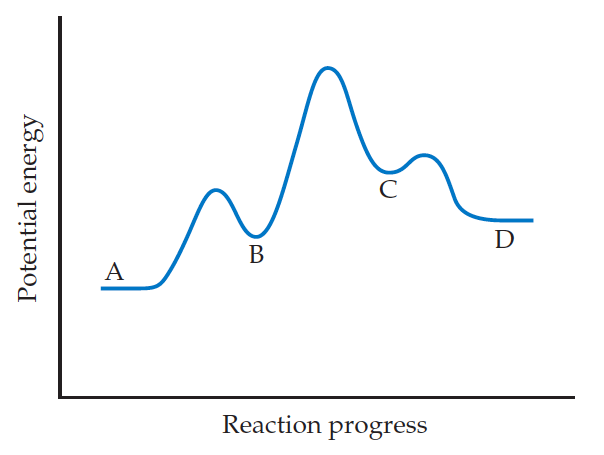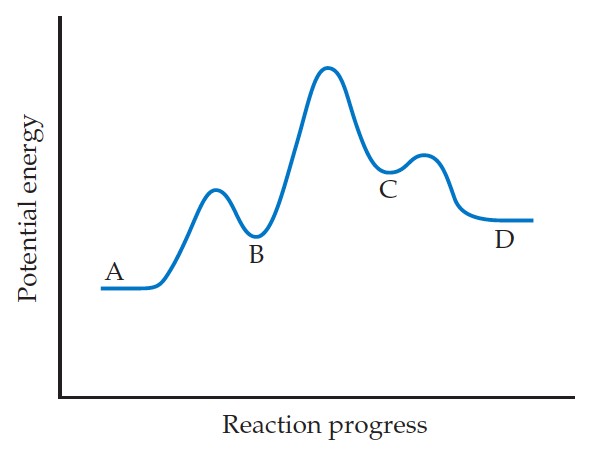Problem: For the reaction A → D, is ΔE positive, negative, or zero?

FREE Expert Solution

We’re being asked to determine if ΔE is positive, negative, or zero for the given reaction.

A → DRecall that an energy diagram is usually read from left to right

The components of a one-step energy diagram are:

79% (248 ratings)
Problem Details

For the reaction A → D, is ΔE positive, negative, or zero?